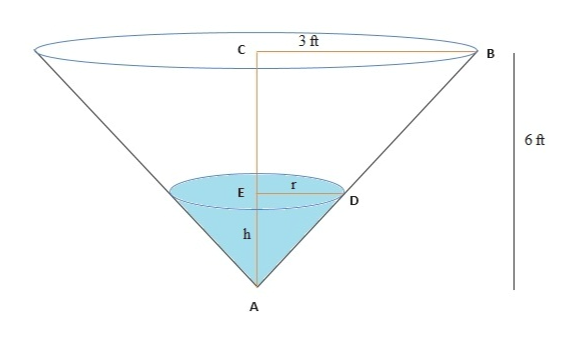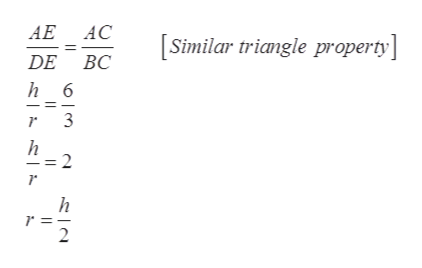An inverted conical water tank with a height o 6 ft and a radius of 3 ft is drained through a hole in the vertex at a rate of 6 ft3/s. What is the rate of change of the water depth when the water depth is 5 ft​?​(Hint​: Use similar​ triangles.)

Question

An inverted conical water tank with a height o 6 ft and a radius of 3 ft is drained through a hole in the vertex at a rate of 6 ft3/s. What is the rate of change of the water depth when the water depth is 5 ft​?​(Hint​: Use similar​ triangles.)

Step 1

Given, height of conical tank 6 ft and radius 3 ft.help_outlineImage Transcriptionclose3 ft С B 6 ft А in ш fullscreen
Step 2

In triangle ABC and triangle ADE,help_outlineImage TranscriptioncloseАЕ АС Similar triangle property] DE BC h 6 3 h = 2 h 2 fullscreen
Step 3

Volume of co...

Want to see the full answer?

See Solution

Want to see this answer and more?

Our solutions are written by experts, many with advanced degrees, and available 24/7

See Solution
Tagged in

Derivative